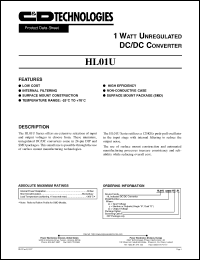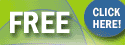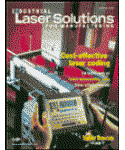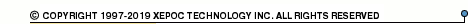More than12 551 964 queries processedHL01U05D05 series datasheets. Manufacturer: CANDD.

 HL01U05D05Y Dual 1 Watt unregulated DC/DC converter. Nom.input voltage 5Vdc, rated output voltage +-5Vdc, rated output current +-100mA. in 24-pin DIP package. Operational temperature range from -40°C to 85°C. Datasheet*) HL01U05D05YH Dual 1 Watt unregulated DC/DC converter. Nom.input voltage 5Vdc, rated output voltage +-5Vdc, rated output current +-100mA. in 24-pin DIP package. Operational temperature range from -40°C to 85°C. Datasheet*) HL01U05D05Z Dual 1 Watt unregulated DC/DC converter. Nom.input voltage 5Vdc, rated output voltage +-5Vdc, rated output current +-100mA. in 24-pin SMD package. Operational temperature range from -40°C to 85°C. Datasheet*) HL01U05D12Y Dual 1 Watt unregulated DC/DC converter. Nom.input voltage 5Vdc, rated output voltage +-12Vdc, rated output current +-41mA. in 24-pin DIP package. Operational temperature range from -40°C to 85°C. Datasheet*) HL01U05D12YH Dual 1 Watt unregulated DC/DC converter. Nom.input voltage 5Vdc, rated output voltage +-12Vdc, rated output current +-41mA. in 24-pin DIP package. Operational temperature range from -40°C to 85°C. Datasheet*) HL01U05D12Z Dual 1 Watt unregulated DC/DC converter. Nom.input voltage 5Vdc, rated output voltage +-12Vdc, rated output current +-41mA. in 24-pin SMD package. Operational temperature range from -40°C to 85°C. Datasheet*) HL01U05D15Y Dual 1 Watt unregulated DC/DC converter. Nom.input voltage 5Vdc, rated output voltage +-15Vdc, rated output current +-33mA. in 24-pin DIP package. Operational temperature range from -40°C to 85°C. Datasheet*) HL01U05D15YH Dual 1 Watt unregulated DC/DC converter. Nom.input voltage 5Vdc, rated output voltage +-15Vdc, rated output current +-33mA. in 24-pin DIP package. Operational temperature range from -40°C to 85°C. Datasheet*) HL01U05D15Z Dual 1 Watt unregulated DC/DC converter. Nom.input voltage 5Vdc, rated output voltage +-15Vdc, rated output current +-33mA. in 24-pin SMD package. Operational temperature range from -40°C to 85°C. Datasheet*) HL01U05S05Y Single 1 Watt unregulated DC/DC converter. Nom.input voltage 5Vdc, rated output voltage 5Vdc, rated output current 200mA. in 24-pin DIP package. Operational temperature range from -40°C to 85°C. Datasheet*) HL01U05S05YH Single 1 Watt unregulated DC/DC converter. Nom.input voltage 5Vdc, rated output voltage 5Vdc, rated output current 200mA. in 24-pin DIP package. Operational temperature range from -40°C to 85°C. Datasheet*) HL01U05S05Z Single 1 Watt unregulated DC/DC converter. Nom.input voltage 5Vdc, rated output voltage 5Vdc, rated output current 200mA. in 24-pin SMD package. Operational temperature range from -40°C to 85°C. Datasheet*) HL01U05S12Y Single 1 Watt unregulated DC/DC converter. Nom.input voltage 5Vdc, rated output voltage 12Vdc, rated output current 83mA. in 24-pin DIP package. Operational temperature range from -40°C to 85°C. Datasheet*) HL01U05S12YH Single 1 Watt unregulated DC/DC converter. Nom.input voltage 5Vdc, rated output voltage 12Vdc, rated output current 83mA. in 24-pin DIP package. Operational temperature range from -40°C to 85°C. Datasheet*) HL01U05S12Z Single 1 Watt unregulated DC/DC converter. Nom.input voltage 5Vdc, rated output voltage 12Vdc, rated output current 83mA. in 24-pin SMD package. Operational temperature range from -40°C to 85°C. Datasheet*) HL01U05S15Y Single 1 Watt unregulated DC/DC converter. Nom.input voltage 5Vdc, rated output voltage 15Vdc, rated output current 67mA. in 24-pin DIP package. Operational temperature range from -40°C to 85°C. Datasheet*) HL01U05S15YH Single 1 Watt unregulated DC/DC converter. Nom.input voltage 5Vdc, rated output voltage 15Vdc, rated output current 67mA. in 24-pin DIP package. Operational temperature range from -40°C to 85°C. Datasheet*) HL01U05S15Z Single 1 Watt unregulated DC/DC converter. Nom.input voltage 5Vdc, rated output voltage 15Vdc, rated output current 67mA. in 24-pin SMD package. Operational temperature range from -40°C to 85°C. Datasheet*) HL01U12D05Y Dual 1 Watt unregulated DC/DC converter. Nom.input voltage 12Vdc, rated output voltage +-5Vdc, rated output current +-100mA. in 24-pin DIP package. Operational temperature range from -40°C to 85°C. Datasheet*) HL01U12D05YH Dual 1 Watt unregulated DC/DC converter. Nom.input voltage 12Vdc, rated output voltage +-5Vdc, rated output current +-100mA. in 24-pin DIP package. Operational temperature range from -40°C to 85°C. Datasheet*) HL01U12D05Z Dual 1 Watt unregulated DC/DC converter. Nom.input voltage 12Vdc, rated output voltage +-5Vdc, rated output current +-100mA. in 24-pin SMD package. Operational temperature range from -40°C to 85°C. Datasheet*) HL01U12D12Y Dual 1 Watt unregulated DC/DC converter. Nom.input voltage 12Vdc, rated output voltage +-12Vdc, rated output current +-41mA. in 24-pin DIP package. Operational temperature range from -40°C to 85°C. Datasheet*) HL01U12D12YH Dual 1 Watt unregulated DC/DC converter. Nom.input voltage 12Vdc, rated output voltage +-12Vdc, rated output current +-41mA. in 24-pin DIP package. Operational temperature range from -40°C to 85°C. Datasheet*) HL01U12D12Z Dual 1 Watt unregulated DC/DC converter. Nom.input voltage 12Vdc, rated output voltage +-12Vdc, rated output current +-41mA. in 24-pin SMD package. Operational temperature range from -40°C to 85°C. Datasheet*) HL01U12D15Y Dual 1 Watt unregulated DC/DC converter. Nom.input voltage 12Vdc, rated output voltage +-15Vdc, rated output current +-33mA. in 24-pin DIP package. Operational temperature range from -40°C to 85°C. Datasheet*) HL01U12D15YH Dual 1 Watt unregulated DC/DC converter. Nom.input voltage 12Vdc, rated output voltage +-15Vdc, rated output current +-33mA. in 24-pin DIP package. Operational temperature range from -40°C to 85°C. Datasheet*) HL01U12D15Z Dual 1 Watt unregulated DC/DC converter. Nom.input voltage 12Vdc, rated output voltage +-15Vdc, rated output current +-33mA. in 24-pin SMD package. Operational temperature range from -40°C to 85°C. Datasheet*) HL01U12S05Y Single 1 Watt unregulated DC/DC converter. Nom.input voltage 12Vdc, rated output voltage 5Vdc, rated output current 200mA. in 24-pin DIP package. Operational temperature range from -40°C to 85°C. Datasheet*) HL01U12S05YH Single 1 Watt unregulated DC/DC converter. Nom.input voltage 12Vdc, rated output voltage 5Vdc, rated output current 200mA. in 24-pin DIP package. Operational temperature range from -40°C to 85°C. Datasheet*) HL01U12S05Z Single 1 Watt unregulated DC/DC converter. Nom.input voltage 12Vdc, rated output voltage 5Vdc, rated output current 200mA. in 24-pin SMD package. Operational temperature range from -40°C to 85°C. Datasheet*) HL01U12S12Y Single 1 Watt unregulated DC/DC converter. Nom.input voltage 12Vdc, rated output voltage 12Vdc, rated output current 83mA. in 24-pin DIP package. Operational temperature range from -40°C to 85°C. Datasheet*) HL01U12S12YH Single 1 Watt unregulated DC/DC converter. Nom.input voltage 12Vdc, rated output voltage 12Vdc, rated output current 83mA. in 24-pin DIP package. Operational temperature range from -40°C to 85°C. Datasheet*) HL01U12S12Z Single 1 Watt unregulated DC/DC converter. Nom.input voltage 12Vdc, rated output voltage 12Vdc, rated output current 83mA. in 24-pin SMD package. Operational temperature range from -40°C to 85°C. Datasheet*) HL01U12S15Y Single 1 Watt unregulated DC/DC converter. Nom.input voltage 12Vdc, rated output voltage 15Vdc, rated output current 67mA. in 24-pin DIP package. Operational temperature range from -40°C to 85°C. Datasheet*) HL01U12S15YH Single 1 Watt unregulated DC/DC converter. Nom.input voltage 12Vdc, rated output voltage 15Vdc, rated output current 67mA. in 24-pin DIP package. Operational temperature range from -40°C to 85°C. Datasheet*) HL01U12S15Z Single 1 Watt unregulated DC/DC converter. Nom.input voltage 12Vdc, rated output voltage 15Vdc, rated output current 67mA. in 24-pin SMD package. Operational temperature range from -40°C to 85°C. Datasheet*) HL01U15D05Y Dual 1 Watt unregulated DC/DC converter. Nom.input voltage 15Vdc, rated output voltage +-5Vdc, rated output current +-100mA. in 24-pin DIP package. Operational temperature range from -40°C to 85°C. Datasheet*) HL01U15D05YH Dual 1 Watt unregulated DC/DC converter. Nom.input voltage 15Vdc, rated output voltage +-5Vdc, rated output current +-100mA. in 24-pin DIP package. Operational temperature range from -40°C to 85°C. Datasheet*) HL01U15D05Z Dual 1 Watt unregulated DC/DC converter. Nom.input voltage 15Vdc, rated output voltage +-5Vdc, rated output current +-100mA. in 24-pin SMD package. Operational temperature range from -40°C to 85°C. Datasheet*) HL01U15D12Y Dual 1 Watt unregulated DC/DC converter. Nom.input voltage 15Vdc, rated output voltage +-12Vdc, rated output current +-41mA. in 24-pin DIP package. Operational temperature range from -40°C to 85°C. Datasheet*) HL01U15D12YH Dual 1 Watt unregulated DC/DC converter. Nom.input voltage 15Vdc, rated output voltage +-12Vdc, rated output current +-41mA. in 24-pin DIP package. Operational temperature range from -40°C to 85°C. Datasheet*) HL01U15D12Z Dual 1 Watt unregulated DC/DC converter. Nom.input voltage 15Vdc, rated output voltage +-12Vdc, rated output current +-41mA. in 24-pin SMD package. Operational temperature range from -40°C to 85°C. Datasheet*) HL01U15D15Y Dual 1 Watt unregulated DC/DC converter. Nom.input voltage 15Vdc, rated output voltage +-15Vdc, rated output current +-33mA. in 24-pin DIP package. Operational temperature range from -40°C to 85°C. Datasheet*) HL01U15D15YH Dual 1 Watt unregulated DC/DC converter. Nom.input voltage 15Vdc, rated output voltage +-15Vdc, rated output current +-33mA. in 24-pin DIP package. Operational temperature range from -40°C to 85°C. Datasheet*) HL01U15D15Z Dual 1 Watt unregulated DC/DC converter. Nom.input voltage 15Vdc, rated output voltage +-15Vdc, rated output current +-33mA. in 24-pin SMD package. Operational temperature range from -40°C to 85°C. Datasheet*) HL01U15S05Y Single 1 Watt unregulated DC/DC converter. Nom.input voltage 15Vdc, rated output voltage 5Vdc, rated output current 200mA. in 24-pin DIP package. Operational temperature range from -40°C to 85°C. Datasheet*) HL01U15S05YH Single 1 Watt unregulated DC/DC converter. Nom.input voltage 15Vdc, rated output voltage 5Vdc, rated output current 200mA. in 24-pin DIP package. Operational temperature range from -40°C to 85°C. Datasheet*) HL01U15S05Z Single 1 Watt unregulated DC/DC converter. Nom.input voltage 15Vdc, rated output voltage 5Vdc, rated output current 200mA. in 24-pin SMD package. Operational temperature range from -40°C to 85°C. Datasheet*) HL01U15S12Y Single 1 Watt unregulated DC/DC converter. Nom.input voltage 15Vdc, rated output voltage 12Vdc, rated output current 83mA. in 24-pin DIP package. Operational temperature range from -40°C to 85°C. Datasheet*) HL01U15S12YH Single 1 Watt unregulated DC/DC converter. Nom.input voltage 15Vdc, rated output voltage 12Vdc, rated output current 83mA. in 24-pin DIP package. Operational temperature range from -40°C to 85°C. Datasheet*) HL01U15S12Z Single 1 Watt unregulated DC/DC converter. Nom.input voltage 15Vdc, rated output voltage 12Vdc, rated output current 83mA. in 24-pin SMD package. Operational temperature range from -40°C to 85°C. Datasheet*) HL01U15S15Y Single 1 Watt unregulated DC/DC converter. Nom.input voltage 15Vdc, rated output voltage 15Vdc, rated output current 67mA. in 24-pin DIP package. Operational temperature range from -40°C to 85°C. Datasheet*) HL01U15S15YH Single 1 Watt unregulated DC/DC converter. Nom.input voltage 15Vdc, rated output voltage 15Vdc, rated output current 67mA. in 24-pin DIP package. Operational temperature range from -40°C to 85°C. Datasheet*) HL01U15S15Z Single 1 Watt unregulated DC/DC converter. Nom.input voltage 15Vdc, rated output voltage 15Vdc, rated output current 67mA. in 24-pin SMD package. Operational temperature range from -40°C to 85°C. Datasheet*) HL01U24D05Y Dual 1 Watt unregulated DC/DC converter. Nom.input voltage 24Vdc, rated output voltage +-5Vdc, rated output current +-100mA. in 24-pin DIP package. Operational temperature range from -40°C to 85°C. Datasheet*) HL01U24D05YH Dual 1 Watt unregulated DC/DC converter. Nom.input voltage 24Vdc, rated output voltage +-5Vdc, rated output current +-100mA. in 24-pin DIP package. Operational temperature range from -40°C to 85°C. Datasheet*) HL01U24D05Z Dual 1 Watt unregulated DC/DC converter. Nom.input voltage 24Vdc, rated output voltage +-5Vdc, rated output current +-100mA. in 24-pin SMD package. Operational temperature range from -40°C to 85°C. Datasheet*) HL01U24D12Y Dual 1 Watt unregulated DC/DC converter. Nom.input voltage 24Vdc, rated output voltage +-12Vdc, rated output current +-41mA. in 24-pin DIP package. Operational temperature range from -40°C to 85°C. Datasheet*) HL01U24D12YH Dual 1 Watt unregulated DC/DC converter. Nom.input voltage 24Vdc, rated output voltage +-12Vdc, rated output current +-41mA. in 24-pin DIP package. Operational temperature range from -40°C to 85°C. Datasheet*) HL01U24D12Z Dual 1 Watt unregulated DC/DC converter. Nom.input voltage 24Vdc, rated output voltage +-12Vdc, rated output current +-41mA. in 24-pin SMD package. Operational temperature range from -40°C to 85°C. Datasheet*) HL01U24D15Y Dual 1 Watt unregulated DC/DC converter. Nom.input voltage 24Vdc, rated output voltage +-15Vdc, rated output current +-33mA. in 24-pin DIP package. Operational temperature range from -40°C to 85°C. Datasheet*) HL01U24D15YH Dual 1 Watt unregulated DC/DC converter. Nom.input voltage 24Vdc, rated output voltage +-15Vdc, rated output current +-33mA. in 24-pin DIP package. Operational temperature range from -40°C to 85°C. Datasheet*) HL01U24D15Z Dual 1 Watt unregulated DC/DC converter. Nom.input voltage 24Vdc, rated output voltage +-15Vdc, rated output current +-33mA. in 24-pin SMD package. Operational temperature range from -40°C to 85°C. Datasheet*) HL01U24S05Y Single 1 Watt unregulated DC/DC converter. Nom.input voltage 24Vdc, rated output voltage 5Vdc, rated output current 200mA. in 24-pin DIP package. Operational temperature range from -40°C to 85°C. Datasheet*) HL01U24S05YH Single 1 Watt unregulated DC/DC converter. Nom.input voltage 24Vdc, rated output voltage 5Vdc, rated output current 200mA. in 24-pin DIP package. Operational temperature range from -40°C to 85°C. Datasheet*) HL01U24S05Z Single 1 Watt unregulated DC/DC converter. Nom.input voltage 24Vdc, rated output voltage 5Vdc, rated output current 200mA. in 24-pin SMD package. Operational temperature range from -40°C to 85°C. Datasheet*) HL01U24S12Y Single 1 Watt unregulated DC/DC converter. Nom.input voltage 24Vdc, rated output voltage 12Vdc, rated output current 83mA. in 24-pin DIP package. Operational temperature range from -40°C to 85°C. Datasheet*) HL01U24S12YH Single 1 Watt unregulated DC/DC converter. Nom.input voltage 24Vdc, rated output voltage 12Vdc, rated output current 83mA. in 24-pin DIP package. Operational temperature range from -40°C to 85°C. Datasheet*) HL01U24S12Z Single 1 Watt unregulated DC/DC converter. Nom.input voltage 24Vdc, rated output voltage 12Vdc, rated output current 83mA. in 24-pin SMD package. Operational temperature range from -40°C to 85°C. Datasheet*) HL01U24S15Y Single 1 Watt unregulated DC/DC converter. Nom.input voltage 24Vdc, rated output voltage 15Vdc, rated output current 67mA. in 24-pin DIP package. Operational temperature range from -40°C to 85°C. Datasheet*) HL01U24S15YH Single 1 Watt unregulated DC/DC converter. Nom.input voltage 24Vdc, rated output voltage 15Vdc, rated output current 67mA. in 24-pin DIP package. Operational temperature range from -40°C to 85°C. Datasheet*) HL01U24S15Z Single 1 Watt unregulated DC/DC converter. Nom.input voltage 24Vdc, rated output voltage 15Vdc, rated output current 67mA. in 24-pin SMD package. Operational temperature range from -40°C to 85°C. Datasheet*) HL01U 1 WATT UNREGULATED DC/DC CONVERTER Datasheet*) HL01U05D05 1 WATT UNREGULATED DC/DC CONVERTER Datasheet*) HL01U05D12 1 WATT UNREGULATED DC/DC CONVERTER Datasheet*) HL01U05D15 1 WATT UNREGULATED DC/DC CONVERTER Datasheet*) HL01U05S05 1 WATT UNREGULATED DC/DC CONVERTER Datasheet*) HL01U05S12 1 WATT UNREGULATED DC/DC CONVERTER Datasheet*) HL01U05S15 1 WATT UNREGULATED DC/DC CONVERTER Datasheet*) HL01U12D05 1 WATT UNREGULATED DC/DC CONVERTER Datasheet*) HL01U12D12 1 WATT UNREGULATED DC/DC CONVERTER Datasheet*) HL01U12D15 1 WATT UNREGULATED DC/DC CONVERTER Datasheet*) HL01U12S05 1 WATT UNREGULATED DC/DC CONVERTER Datasheet*) HL01U12S12 1 WATT UNREGULATED DC/DC CONVERTER Datasheet*) HL01U12S15 1 WATT UNREGULATED DC/DC CONVERTER Datasheet*) HL01U15D05 1 WATT UNREGULATED DC/DC CONVERTER Datasheet*) HL01U15D12 1 WATT UNREGULATED DC/DC CONVERTER Datasheet*) HL01U15D15 1 WATT UNREGULATED DC/DC CONVERTER Datasheet*) HL01U15S05 1 WATT UNREGULATED DC/DC CONVERTER Datasheet*) HL01U15S12 1 WATT UNREGULATED DC/DC CONVERTER Datasheet*) HL01U15S15 1 WATT UNREGULATED DC/DC CONVERTER Datasheet*) HL01U24D05 1 WATT UNREGULATED DC/DC CONVERTER Datasheet*) HL01U24D12 1 WATT UNREGULATED DC/DC CONVERTER Datasheet*) HL01U24D15 1 WATT UNREGULATED DC/DC CONVERTER Datasheet*) HL01U24S05 1 WATT UNREGULATED DC/DC CONVERTER Datasheet*) HL01U24S12 1 WATT UNREGULATED DC/DC CONVERTER Datasheet*) HL01U24S15 1 WATT UNREGULATED DC/DC CONVERTER Datasheet*)Industrial Laser Solutions for Manufacturing Magazine.Subscribe now for FREE !